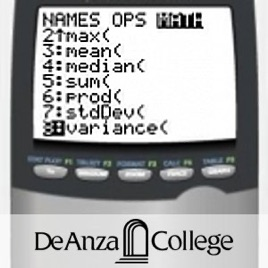14エピソード

Introduction to data analysis making use of graphical and numerical techniques to study patterns and departures from patterns. The student studies randomness with an emphasis on understanding variation, collects information in the face of uncertainty, checks distributional assumptions, tests hypotheses, uses probability as a tool for anticipating what the distribution of data may look like under a set of assumptions, and uses appropriate statistical models to draw conclusions from data. The course introduces the student to applications in engineering, business, economics, medicine, education, the sciences, and other related fields. The use of technology (computers or graphing calculators) will be required in certain applications.Math 10: Elementary Statistics and Probability - Without Captions De Anza College

• 教育

Introduction to data analysis making use of graphical and numerical techniques to study patterns and departures from patterns. The student studies randomness with an emphasis on understanding variation, collects information in the face of uncertainty, checks distributional assumptions, tests hypotheses, uses probability as a tool for anticipating what the distribution of data may look like under a set of assumptions, and uses appropriate statistical models to draw conclusions from data. The course introduces the student to applications in engineering, business, economics, medicine, education, the sciences, and other related fields. The use of technology (computers or graphing calculators) will be required in certain applications.